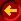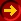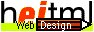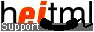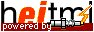## regexcomp, regexexec, createMatchArray

 Format: regexcomp ( pattern, flags )
 Format: regexexec ( regex, string, matcharray, [position, [flags]] )
 Format: createMatchArray ( size )

Purpose: These functions provide access to the regular expression library pcre (1 2). Using regexcomp() a regular expression pattern can be compiled for later use. regexexec() uses a compiled regular expression (maybe several times) to match strings to this pattern. The exact syntax and semantics of the regular expressions is documented with the pcre library.

The regexexec() function returns an array of matching positions. It contains start and end positions for each parenthesized subexpressions within the regular expression. This array must be created before the first call with the createMatchArray() function.

Return Values: When successful, regexcomp() returns a compiled representation of the regular expression pattern (type tPcre). If regexcomp() fails, the compilation aborts with an error message.

regexexec() returns an integer denoting how many elements of matcharray are used if the regular expression matches the string, leaving the matching positions in matcharray, and returns null if not.

createMatchArray() returns a tuple of tuples. There are two array tuples, each of size size. The two arrays are fields of the main tuple with fieldnames start and end. The array fields of both arrays are initialized with integer 0s. The regexexec() function puts start position of each parenthesized subexpression into the start array and the corresponding end positions into the end array.

Position Argument:

The position argument must be an integer variable denoting the position in the string where the search starts.

Flags Argument:

The functions regexcomp() and regexexec() have an additional argument flags. It must be a string containing a comma or white space seperated list of flags. The following flags are supported and are documented in the pcre documentation.

ANCHORED, BSR_ANYCRLF, BSR_UNICODE, CASELESS, DOLLAR_ENDONLY, DOTALL, DUPNAMES, EXTENDED, EXTRA, FIRSTLINE, JAVASCRIPT_COMPAT, MULTILINE, NEWLINE_CR, NEWLINE_LF, NEWLINE_CRLF, NEWLINE_ANYCRLF, NEWLINE_ANY, NO_AUTO_CAPTURE, NO_UTF8_CHECK, UNGREEDY, UTF8

ANCHORED, NEWLINE_CR, NEWLINE_LF, NEWLINE_CRLF, NEWLINE_ANYCRLF, NEWLINE_ANY, NOTBOL, NOTEOL, NOTEMPTY, NO_START_OPTIMIZE, NO_UTF8_CHECK, PARTIAL

Example:

 heitml input: ``` ``` resulting output: ``` start=(=0, =0, =5, =13, =null) end=(=19, =4, =12, =19, =null) (mailboxname="test", hostname="radpage", topleveldomain="nospam") ```

Example:

 heitml input: resulting output: ``` 1st no match 2nd no match``` ``` start=(=0, =0, =0, =3, =9, =9) end=(=11, =9, =3, =9, =11, =11) 2nd no match```

Example:

 heitml input: resulting output: ``` "; pos=m.end; n = regexexec (re, s, m, pos); /while; > ``` ``` HelloThistestprintsallWordsnocharsandnonumbers ```

Example:

 heitml input: resulting output: ``` ])"); m = createMatchArray(4); pos = 0; n = regexexec (re, s, m, pos); while !isnull(n); if !isnull(m.start) ><\br>Word: < elsif !isnull(m.start) ><\br>Number: < elsif !isnull(m.start) ><\br>Punctation: < /if; ? substring(s,m.start,m.end); ? " "; pos=m.end; n = regexexec (re, s, m, pos); /while > ``` ``` Word: Hello Punctation: ! Word: This Number: 55 Word: test Word: prints Word: all Word: Tokens Punctation: Punctation: ! Punctation: + Word: and Number: 34 Word: numbers ```

This page was dynamically generated by the web application development tool RADpage of H.E.I. See "Was ist AJAX? " (in German). In Germany H.E.I. provides Webdesign in Mannheim and Web Programming (Programmierung).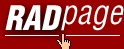Homepage Intro/Features Component Guide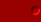Programming Language Guide Language Ref. General Design Lexical Structure Expressions Objects Methods Classes heitml Tags heitml Functions Advanced Functions containsRE containsCaseRE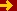createMatchArray fileio_chmod fileio_fclose fileio_feof fileio_fopen fileio_fread fileio_fwrite fileio_strerror indexRE indexCaseREregexcompregexexec request_string request_tuple substr substRE substallRE TuSearch TuSearchTuple TuSort Database Access Global Variables Form Fields Server Variables Sessions heitml Syntax Component Ref. Class Library User Components Tutorial New Features heitml 1User Guide Services Privacy DatenschutzContact / Impressum